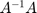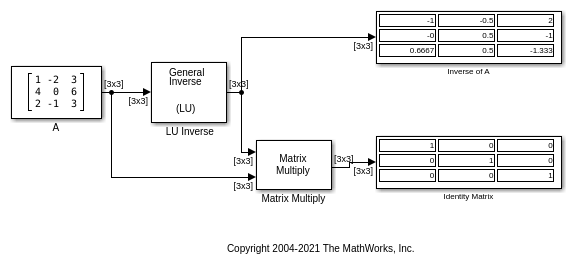# Find Inverse of Matrix Using the LU Inverse Block

Compute the inverse of a 3-by-3 square matrixusing the LU Inverse block. Multiply the input matrixwith the computed inverseusing the Matrix Multiply block. Verify that the productforms an identity matrix.

Open and run the model. The output of the Matrix Multiply block yields the identity matrix of order 3, as expected.